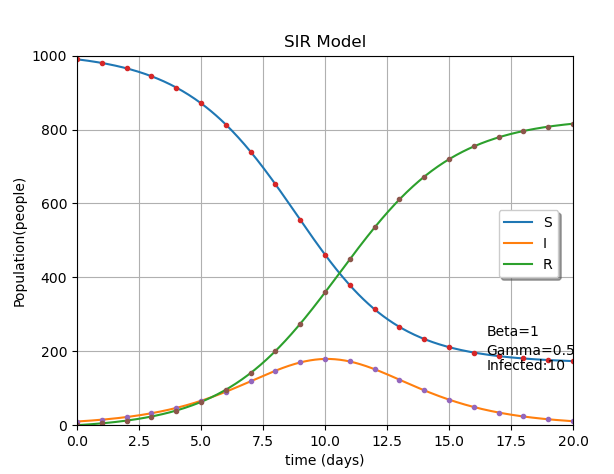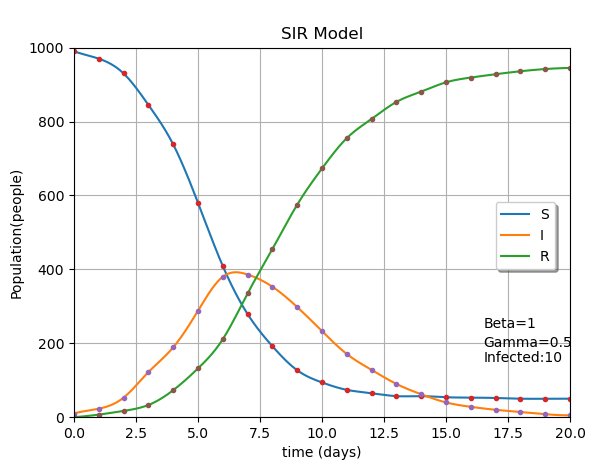## ¶ 2. 數學定義

### ¶ 2.1 SIR 模型定義

$$\frac{dS}{dt} = - \frac{βIS}{N}$$

$$\frac{dI}{dt} = \frac{βIS}{N} - 𝛾I$$

$$\frac{dR}{dt} = 𝛾I$$

$$\frac{dS}{dt} + \frac{dI}{dt} + \frac{dR}{dt} = 0$$
$$S(t) + T(t) + R(t) = N$$

### ¶ 2.2 基本傳染數 $R_0$

$R_0$ 的定義如下：

$$R_0 = 𝜏 × 𝑐̅ × d = \frac{β}{𝛾}$$

$$β = 𝜏 × 𝑐̅$$
$$γ = d^{-1}$$

$$\frac{βIS}{N} - 𝛾I > 0$$
$$\frac{βIS}{N} > 𝛾I$$

$$\frac{β}{𝛾} = R_0 > 1$$

### ¶ 2.3 指數分布（Exponential Distribution）

\begin{align} f(x; \lambda) &= 1 - e ^ {- \lambda x}&, x>=0 \\ &= 0 &, x<0 \\ \end{align}

## ¶ 3 程式模擬

### ¶ 3.1 巨觀模擬

def SIR(S, I, R):

# 計算變化
dS = - Beta * S *  I/ N
dR = Gamma * I
dI = - dS - dR

# 把變化加回去
s = S + dS
i = I + dI
r = R + dR

# 邊界檢查
if (s < 0):
s = 0
if (i > N):
i = N
if (r > N):
r = N

return s, i, r### ¶ 3.2 微觀模擬

class Person:
def __init__(self, id, state):
self.id = id
self.state = state

def step(self, people, dt=1):
if self.state == State.SUS:
new_beta = 0

# 假設和所有人都碰過, people 是所有人的 List
for person in people:
if person.state == State.INF:
new_beta += Beta

if InfectiousEvent(new_beta, dt):
self.state = State.INF

elif self.state == State.INF:
if RecoveredEvent(Gamma, dt):
self.state = State.RECV


step() 就是每一回合和所有人接觸會怎樣，如果這個人是健康的（State.SUS）的話，就讓他和其他人互動，並算出最後的感染力，並算出會感染的機率。如果這個人已經被感染（State.INF），那就算她康復的機率。如果這人已經康復（State.RECV），在此模型中康復者不會復發，因此不處理。

# 感染的事件
def InfectiousEvent(rate, dt):
try:
return -log(random()) / rate*k < dt
except ZeroDivisionError:
return 0

# 恢復的事件
def RecoveredEvent(rate, dt):
try:
return -log(random()) / rate*h < dt
except ZeroDivisionError:
return 0## ¶ 4 結論

SIR 模型或機率模型都只是一種簡化假設模型，他們可能可以解釋某個傳染病的擴散，也可能無法解釋。每個模型都有適合的時空背景，例如人口是否封閉，是否可以透過家禽傳染，是否有實施居家隔離政策等等。我們可以藉由建立符合當下背景的模型，透過模擬結果一窺傳染病的影響，例如是否會造成大流行，藉此做到公共衛生預防和因應。